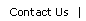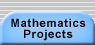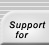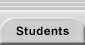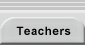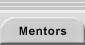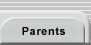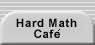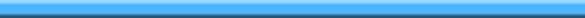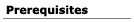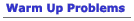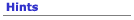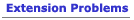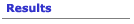## Prerequisites for Marion Walter's Theorem

There are several ways to approach the problem and your approach will probably depend on which mathematical topics you are familiar with.

- You can use classical geometry.  For that, you'll need  a solid background in proportionality, areas of polygons, and similarity.
- You can use analytic geometry.  You'll need to know how to find equations of lines and how to solve linear systems.
- You could use vector geometry. You'll need to know how to find vector equations of lines  and how to use determinants to find areas.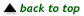Translations of mathematical formulas for web display were created by tex4ht. © Copyright 2003 Education Development Center, Inc. (EDC)